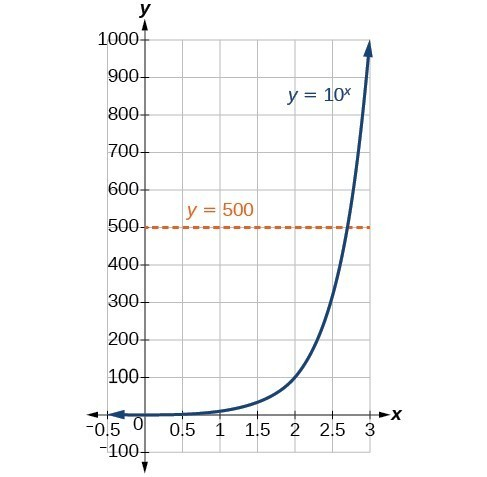## Defining Logarithmic Functions

### Learning Outcomes

• Convert from logarithmic form to exponential form
• Convert from exponential form to logarithmic form

In order to analyze the magnitude of earthquakes or compare the magnitudes of two different earthquakes, we need to be able to convert between logarithmic and exponential form. For example, suppose the amount of energy released from one earthquake were $500$ times greater than the amount of energy released from another. We want to calculate the difference in magnitude. The equation that represents this problem is ${10}^{x}=500$, where $x$ represents the difference in magnitudes on the Richter Scale. How would we solve for $x$?

We have not yet learned a method for solving exponential equations. None of the algebraic tools discussed so far is sufficient to solve ${10}^{x}=500$. We know that ${10}^{2}=100$ and ${10}^{3}=1000$, so it is clear that $x$ must be some value between $2$ and $3$, since $y={10}^{x}$ is increasing. We can examine a graph to better estimate the solution.Estimating from a graph, however, is imprecise. To find an algebraic solution, we must introduce a new function. Observe that the graph above passes the horizontal line test. The exponential function $y={b}^{x}$ is one-to-one, so its inverse, $x={b}^{y}$ is also a function. As is the case with all inverse functions, we simply interchange x and y and solve for y to find the inverse function. To represent y as a function of x, we use a logarithmic function of the form $y={\mathrm{log}}_{b}\left(x\right)$. The base b logarithm of a number is the exponent by which we must raise b to get that number.

We read a logarithmic expression as, “The logarithm with base b of x is equal to y,” or, simplified, “log base b of x is y.” We can also say, “b raised to the power of y is x,” because logs are exponents. For example, the base $2$ logarithm of $32$ is $5$, because $5$ is the exponent we must apply to $2$ to get $32$. Since ${2}^{5}=32$, we can write ${\mathrm{log}}_{2}32=5$. We read this as “log base $2$ of $32$ is $5$.”

We can express the relationship between logarithmic form and its corresponding exponential form as follows:

${\mathrm{log}}_{b}\left(x\right)=y\Leftrightarrow {b}^{y}=x,\text{}b>0,b\ne 1$

Note that the base b is always positive.Because logarithms are functions, they are most correctly written as ${\mathrm{log}}_{b}\left(x\right)$, using parentheses to denote function evaluation, just as we would with $f\left(x\right)$. However, when the input is a single variable or number, it is common to see the parentheses dropped and the expression written without parentheses, as ${\mathrm{log}}_{b}x$. Note that many calculators require parentheses around the x.

The parentheses in a logarithmic expression may contain a single variable like x, or it may contain another expression like 2x-3.  We refer to the contents inside of the parentheses as the argument.  For example, in the function $y={\mathrm{log}}_{b}\left(x\right)$, we say that x is the argument.  In the function $y={\mathrm{log}}_{b}\left(2x-3\right)$, the argument is $2x-3$.

We can illustrate the notation of logarithms as follows:Notice that, comparing the logarithm function and the exponential function, the input and the output are switched. This means $y={\mathrm{log}}_{b}\left(x\right)$ and $y={b}^{x}$ are inverse functions.

### Definition of the Logarithmic Function

A logarithm with base b of a positive number x satisfies the following definition.

For $x>0,b>0,b\ne 1$,

$y={\mathrm{log}}_{b}\left(x\right)\text{ is the same as }{b}^{y}=x$

where

• we read ${\mathrm{log}}_{b}\left(x\right)$ as, “the logarithm with base b of x” or the “log base b of x.”
• the logarithm y is the exponent to which b must be raised to get x.
• The input x is referred to as the argument of the logarithm.

Also, since the logarithmic and exponential functions switch the x and y values, the domain and range of the exponential function are interchanged for the logarithmic function. Therefore,

• the domain of the logarithm function with base $b \text{ is} \left(0,\infty \right)$.
• the range of the logarithm function with base $b \text{ is} \left(-\infty ,\infty \right)$.

In our first example, we will convert logarithmic equations into exponential equations.

### Example

Write the following logarithmic equations in exponential form.

1. ${\mathrm{log}}_{6}\left(\sqrt{6}\right)=\frac{1}{2}$
2. ${\mathrm{log}}_{3}\left(9\right)=2$

In the following video, we present more examples of rewriting logarithmic equations as exponential equations.

### How To: Given an equation in logarithmic form ${\mathrm{log}}_{b}\left(x\right)=y$, convert it to exponential form

1. Examine the equation $y={\mathrm{log}}_{b}x$ and identify b, y, and x.
2. Rewrite ${\mathrm{log}}_{b}x=y$ as ${b}^{y}=x$.

Can we take the logarithm of a negative number? Re-read the definition of a logarithm and formulate an answer.  Think about the behavior of exponents.  You can use the textbox below to formulate your ideas before you look at an answer.

## Convert from Exponential to Logarithmic Form

To convert from exponential form to logarithmic form, we follow the same steps in reverse. We identify the base b, exponent x, and output y. Then we write $x={\mathrm{log}}_{b}\left(y\right)$.

### Example

Write the following exponential equations in logarithmic form.

1. ${2}^{3}=8$
2. ${5}^{2}=25$
3. ${10}^{-4}=\frac{1}{10,000}$

In our last video, we show more examples of writing logarithmic equations as exponential equations.

## Summary

The base b logarithm of a number is the exponent by which we must raise b to get that number. Logarithmic functions are the inverse of exponential functions, and it is often easier to understand them through this lens. We can never take the logarithm of a negative number, therefore ${\mathrm{log}}_{b}\left(x\right)=y$ is defined for $b>0$

## Contribute!

Did you have an idea for improving this content? We’d love your input.